# Series Resonance Notes | Study Network Theory (Electric Circuits) - Electrical Engineering (EE)

## Electrical Engineering (EE): Series Resonance Notes | Study Network Theory (Electric Circuits) - Electrical Engineering (EE)

The document Series Resonance Notes | Study Network Theory (Electric Circuits) - Electrical Engineering (EE) is a part of the Electrical Engineering (EE) Course Network Theory (Electric Circuits).
All you need of Electrical Engineering (EE) at this link: Electrical Engineering (EE)

Series Resonance Circuit Diagram
If the resonance occurs in series RLC circuit, then it is called as Series Resonance. Consider the following series RLC circuit, which is represented in phasor domain.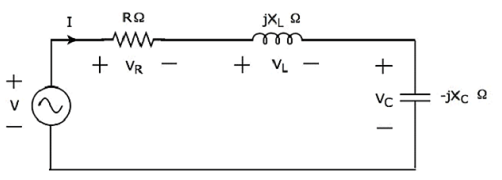Here, the passive elements such as resistor, inductor and capacitor are connected in series. This entire combination is in series with the input sinusoidal voltage source.
Apply KVL around the loop.
V−VR−VL−V= 0
⇒ V−IR−I(jXL)−I(−jXC) = 0
⇒ V = IR+I(jXL)+I(−jXC)
⇒ V = I[R+j(XL−XC)]  Equation 1
The above equation is in the form of V = IZ.
Therefore, the impedance Z of series RLC circuit will be
Z = R+j(XL−XC)

Parameters & Electrical Quantities at Resonance
Now, let us derive the values of parameters and electrical quantities at resonance of series RLC circuit one by one.

Resonant Frequency
The frequency at which resonance occurs is called as resonant frequency fr. In series RLC circuit resonance occurs, when the imaginary term of impedance Z is zero, i.e., the value of XL − XC should be equal to zero.
⇒ XL = XC
Substitute XL = 2πfL and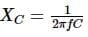in the above equation.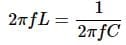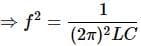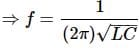Therefore, the resonant frequency fr of series RLC circuit is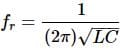Where, L is the inductance of an inductor and C is the capacitance of a capacitor.
The resonant frequency fr of series RLC circuit depends only on the inductance L and capacitance C. But, it is independent of resistance R.

Impedance
We got the impedance Z of series RLC circuit as
Z = R+j(XL − XC)
Substitute XL = XC in the above equation.
Z = R+j(XC − XC)
⇒ Z = R+j(0)
⇒ Z = R
At resonance, the impedance Z of series RLC circuit is equal to the value of resistance R, i.e., Z = R.

Current flowing through the Circuit
Substitute XL − XC = 0 in Equation 1
V = I[R+j(0)]
⇒ V = IR
⇒ I = V/R
Therefore, current flowing through series RLC circuit at resonance is I = V/R
At resonance, the impedance of series RLC circuit reaches to minimum value. Hence, the maximum current flows through this circuit at resonance.

Voltage across Resistor
The voltage across resistor is
VR = IR
Substitute the value of I in the above equation.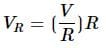⇒ VR = V
Therefore, the voltage across resistor at resonance is VR = V.

Voltage across Inductor
The voltage across inductor is
VL = I(jXL)
Substitute the value of I in the above equation.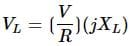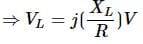⇒ VL = jQV
Therefore, the voltage across inductor at resonance is VL = jQV.
So, the magnitude of voltage across inductor at resonance will be
|VL| = QV
Where Q is the Quality factor and its value is equal to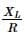Voltage across Capacitor
The voltage across capacitor is
VC = I(−jXC)
Substitute the value of I in the above equation.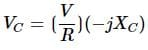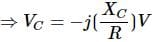⇒ VC = −jQV
Therefore, the voltage across capacitor at resonance is VC = −jQV.
So, the magnitude of voltage across capacitor at resonance will be
|VC| = QV
Where Q is the Quality factor and its value is equal to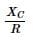Note − Series resonance RLC circuit is called as voltage magnification circuit, because the magnitude of voltage across the inductor and the capacitor is equal to Q times the input sinusoidal voltage V.

The document Series Resonance Notes | Study Network Theory (Electric Circuits) - Electrical Engineering (EE) is a part of the Electrical Engineering (EE) Course Network Theory (Electric Circuits).
All you need of Electrical Engineering (EE) at this link: Electrical Engineering (EE)Use Code STAYHOME200 and get INR 200 additional OFF

## Network Theory (Electric Circuits)

23 videos|21 docs|27 tests

Track your progress, build streaks, highlight & save important lessons and more!

,

,

,

,

,

,

,

,

,

,

,

,

,

,

,

,

,

,

,

,

,

;